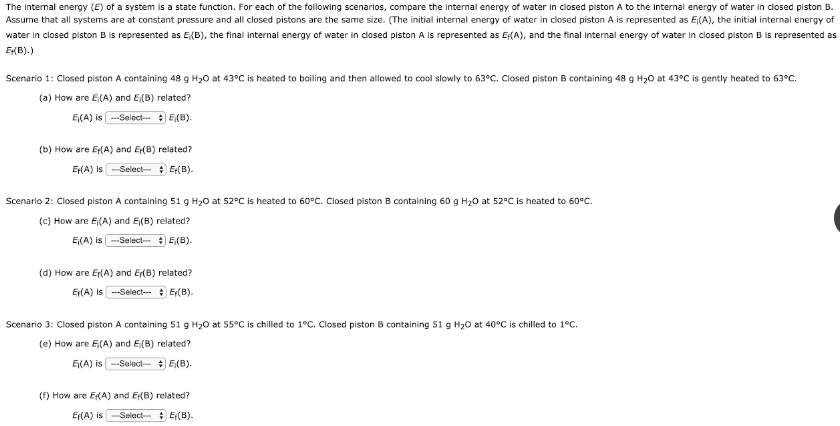# The internal energy (E) of a system is a state function. For each of the following scenarios, compare the internal energy of water in closed piston A to the internal energy of water in closed piston B. Assume that all systems are at constant pressure and all closed pistons are the same size. (The initial internal energy of water in closed piston A is represented as Ei(A), the initial internal energy of water in closed piston B is represented as Ei(B), the final internal energy of water in closed piston A is represented as Ef(A), and the final internal energy of water in closed piston B is represented as Ef(B). Scenario 1: Closed piston A containing 48 g H2O at 43°C is heated to boiling and then allowed to cool slowly to 63°C. Closed piston B containing 48 g H2O at 43°C is gently heated to 63°C. (a) How are Ei(A) and Ei(B) related? (b) How are Ef(A) and Ef(B) related? Scenario 2: Closed piston A containing 51 g H2O at 52°C is heated to 60°C. Closed piston B containing 60 g H2O at 52°C is heated to 60°C. (c) How are Ei(A) and Ei(B) related? (d) How are Ef(A) and Ef(B) related? Scenario 3: Closed piston A containing 51 g H2O at 55°C is chilled to 1°C. Closed piston B containing 51 g H2O at 40°C is chilled to 1°C. (e) How are Ei(A) and Ei(B) related? (f) How are Ef(A) and Ef(B) related?